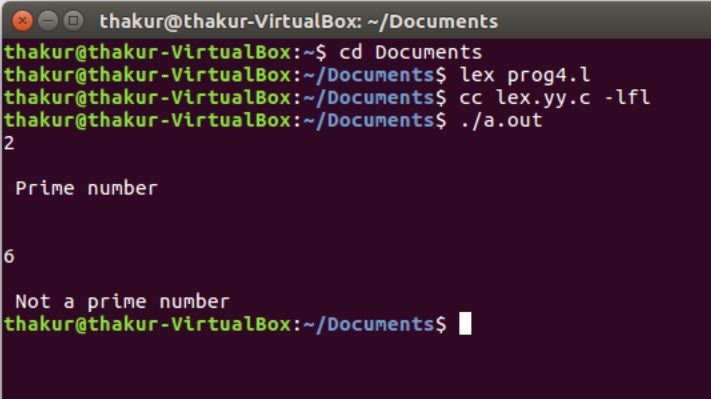# Lex Program to check whether a number is Prime or Not

• Last Updated : 01 May, 2019

Problem: Write a Lex Program to check whether a number is Prime or Not.

Explanation:
Lex is a computer program that generates lexical analyzers and was written by Mike Lesk and Eric Schmidt. Lex reads an input stream specifying the lexical analyzer and outputs source code implementing the lexer in the C programming language.

Definition: A prime number is a whole number greater than 1 whose only factors are 1 and itself. A factor is a whole numbers that can be divided evenly into another number. The first few prime numbers are: 2, 3, 5, 7, 11, 13, 17, 19, 23 and 29.

Examples:

```Input: 2
Output: Prime number

Input: 6
Output: Not a Prime number ```

Implementation:

 `/* Lex Program to check whether a number is Prime or Not */`` ` `%{``   ``/* Definition section */``   ``#include``   ``#include``   ``int` `flag,c,j;``%}`` ` `/* Rule Section */``%%``[0-9]+ {c=``atoi``(yytext);``         ``if``(c==2)``         ``{``           ``printf``(``"\n Prime number"``);``         ``}``         ``else` `if``(c==0 || c==1)``         ``{``           ``printf``(``"\n Not a Prime number"``);``         ``}``         ``else``         ``{``           ``for``(j=2;j

Output:My Personal Notes arrow_drop_up